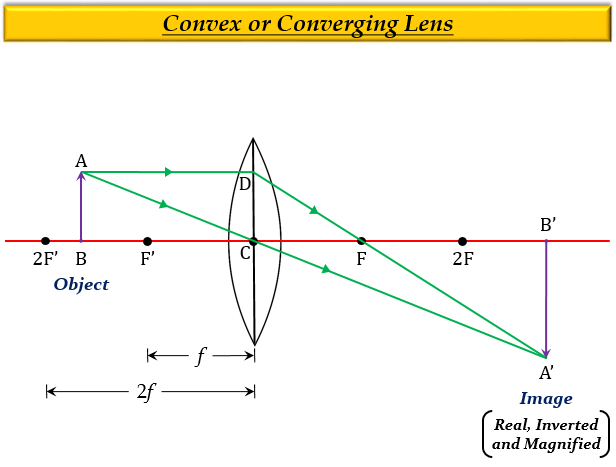# Draw a ray diagram to show the formation of a real magnified image by a convex lens. (In your sketch the position of object and image with respect to the principal focus of lens should be shown clearly).

Formation of a real magnified image by a convex lens when the object is placed between $F'$ and $2F'$ (or between $f$ and $2f$).Explanation

When an object is placed between $F'$ and $2F'$, means the object is at a distance greater than the focal length $f$ and less than twice the focal length $2f$, then the image formed is real, inverted, larger than the object (or magnified), and beyond the $2F$ on the other side of the lens.

Updated on: 10-Oct-2022

80 Views